Theory and Modern Applications

Symmetry discrete-time delayed neural network

Abstract

In this paper, we consider a three-dimensional discrete neural network model with delay. The characteristic equation of the linearized system at the zero solution is a polynomial equation involving very high order terms. We derive some sufficient and necessary conditions on the asymptotic stability and multiple bifurcations of the zero solution. We give computer simulations to support the theoretical predictions.

1 Introduction

Dynamical systems theory plays an important role in many areas of mathematics and physics because it provides the building blocks that allow us to understand the changes many physical systems experience in their dynamics when parameters are varied. In the research of nonlinear dynamical systems, symmetric systems have become an important topic. Symmetric systems appear naturally in many applications. Often the symmetry reflects certain spatial invariant of the dynamical systems. Symmetries change the generic behavior of a dynamical system dramatically, and in recent years, there has been rapid progress in the development of a bifurcation theory for periodic orbits of symmetric dynamical systems; see, e.g., .

Artificial neural network is used to mimic the human brain structure and function. Since 1980s, the theories and applications of neural networks have been greatly developed after the work of Hopfield .

A discrete Hopfield neural network is one of the most interesting topics in spatial extended systems. Recently, the dynamical behaviors (including stability, instability, periodic oscillatory and chaos) of the discrete-time Hopfield neural networks without or with delay have received increasing interest due to their promising potential applications in many fields. Some results have been reported; see .

In this paper, we consider the following three-dimensional discrete Hopfield neural network with delay:

$\left\{\begin{array}{c}{x}_{n+1}=a{x}_{n}+\alpha f\left({x}_{n}\right)+\beta g\left({y}_{n-k}\right),\hfill \\ {y}_{n+1}=a{y}_{n}+\alpha f\left({y}_{n}\right)+\beta g\left({z}_{n-k}\right),\hfill \\ {z}_{n+1}=a{z}_{n}+\alpha f\left({z}_{n}\right)+\beta g\left({x}_{n-k}\right),\hfill \end{array}$
(1.1)

where α, β, a are parameters.

The presence of symmetries changes the nature and type of bifurcations that a dynamical system may undergo. There is a lot of research into equivariant bifurcations of ODEs. We refer the readers to Golubitsky . But, until now, there have been few papers to discuss equivariant bifurcation problems in discrete neural networks, which motivated us to write this paper. The goal of this paper is to investigate how parameters affect the discrete neural network with delay (1.1) by using the symmetric groups theory of Golubitsky .

Accordingly, the paper is organized as follows. In Sections 2 and 3, we show that the structure of the system (1.1) can be represented by a cyclic group ${Z}_{3}$. The generalized center subspace is invariant under the action of the symmetry group and the center manifold reduction can be performed in such a way that the reduced equations commute with the restricted action of the symmetry group. We obtain some important results about spontaneous bifurcations of multiple branches of periodic solutions and their spatio-temporal patterns, which describe the oscillatory mode of each neural cell. We also consider the direction and stability of the Hopf bifurcation in a discrete neural network model. Finally, some numerical simulations are carried out to support the analysis results.

2 ${Z}_{3}$-equivariant and linear stability of a discrete neural network

Assume that ${X}_{n}={\left({x}_{n},{y}_{n},{z}_{n}\right)}^{T}$, then Eq. (1.1) can be rewritten as

$\begin{array}{rcl}{X}_{n+1}& =& \left(\begin{array}{ccc}a& 0& 0\\ 0& a& 0\\ 0& 0& a\end{array}\right){X}_{n}+\left(\begin{array}{ccc}\alpha & 0& 0\\ 0& \alpha & 0\\ 0& 0& \alpha \end{array}\right)\left(\begin{array}{c}f\left({\left({X}_{n}\right)}_{1}\right)\\ f\left({\left({X}_{n}\right)}_{2}\right)\\ f\left({\left({X}_{n}\right)}_{3}\right)\end{array}\right)\\ +\left(\begin{array}{ccc}0& \beta & 0\\ 0& 0& \beta \\ \beta & 0& 0\end{array}\right)\left(\begin{array}{c}g\left({\left({X}_{n-k}\right)}_{1}\right)\\ g\left({\left({X}_{n-k}\right)}_{2}\right)\\ g\left({\left({X}_{n-k}\right)}_{3}\right)\end{array}\right),\end{array}$
(2.1)

where ${\left({X}_{n}\right)}^{T}={\left[{\left({X}_{n}\right)}_{1},{\left({X}_{n}\right)}_{2},{\left({X}_{n}\right)}_{3}\right]}^{T}$. Throughout this section, to establish the main results for Eq. (1.1), we make the following hypothesis on the activation functions in Eq. (1.1):

(H1): $f,g:R⟶R$ are ${C}^{1}$-smooth functions with $f\left(0\right)=0$; ${f}^{\prime }\left(0\right)=1$ and $g\left(0\right)=0$; ${g}^{\prime }\left(0\right)=1$.

Under the assumption (H1), the origin $\left(0,0,0\right)$ is an equilibrium of Eq. (1.1). Linearizing the system (2.1) about origin $\left(0,0,0\right)$ gives the following linear system:

${Y}_{n+1}=\left(\begin{array}{ccc}a+\alpha & 0& 0\\ 0& a+\alpha & 0\\ 0& 0& a+\alpha \end{array}\right){Y}_{n}+\left(\begin{array}{ccc}0& \beta & 0\\ 0& 0& \beta \\ \beta & 0& 0\end{array}\right){Y}_{n-k}.$
(2.2)

For discussing the linear stability and Hopf bifurcations of Eq. (2.1), we need to consider the characteristic equation for Eq. (2.2). We can use the method of Zhang and Zheng  to mark

${M}_{n+1}=A{M}_{n},$

where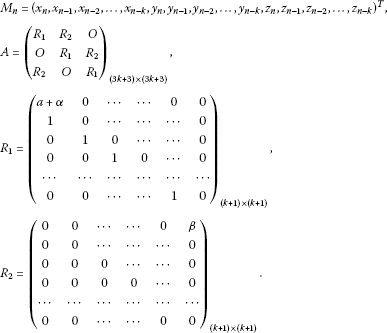Using Lemma 2.1 in Ref. , we can obtain the characteristic equation for Eq. (2.2):

$\begin{array}{rcl}|\lambda E-A|& =& \left[{\lambda }^{k+1}-\left(a+\alpha \right){\lambda }^{k}-\beta \right]\left[{\lambda }^{k+1}-\left(a+\alpha \right){\lambda }^{k}-\beta {e}^{\frac{2\pi i}{3}}\right]\left[{\lambda }^{k+1}-\left(a+\alpha \right){\lambda }^{k}-\beta {e}^{\frac{4\pi i}{3}}\right]\\ =& {\mathrm{\Delta }}_{1}{\mathrm{\Delta }}_{2}{\mathrm{\Delta }}_{3}=0,\end{array}$
(2.3)

where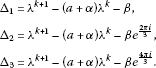It is not difficult to verify that $a+bi$ is a root of ${\mathrm{\Delta }}_{2}=0$ if and only if $a-bi$ is a root of ${\mathrm{\Delta }}_{3}=0$. In order to study the distribution of Eq. (2.2), it is sufficient to investigate ${\mathrm{\Delta }}_{1}=0$, ${\mathrm{\Delta }}_{2}=0$.

In what follows, we denote β as a bifurcating parameter. The analysis of the distribution of roots to Eq. (2.3) is based on the conclusion given in : the sum of the order of zeros of Eq. (2.4) can change only if a zero appears or accesses the unit circle as the parameter β is varied. We make the following assumptions:

(H2): $|a+\alpha |<1$;

(H3): $|\beta |>|1-\left(a+\alpha \right)|$.

Consider ${\mathrm{\Delta }}_{1}=0$. Let ${e}^{i{\omega }_{1}}$ be a root of ${\mathrm{\Delta }}_{1}=0$. Then

${e}^{i\left(k+1\right){\omega }_{1}}-\left(a+\alpha \right){e}^{ik{\omega }_{1}}=\beta .$

That is,

$\left\{\begin{array}{c}cos\left(k+1\right){\omega }_{1}-\left(a+\alpha \right)cosk{\omega }_{1}=\beta ,\hfill \\ sin\left(k+1\right){\omega }_{1}-\left(a+\alpha \right)sink{\omega }_{1}=0.\hfill \end{array}$

So,

$cos{\omega }_{1}=1+\frac{{\left(1-\left(a+\alpha \right)\right)}^{2}-{\beta }^{2}}{2\left(a+\alpha \right)}.$

Hence,

(2.4)

For further analysis, we found that the transversality conditions are met:

$\frac{d{|\lambda |}^{2}}{d\beta }{|}_{\beta ={\beta }_{1j}}=2{\beta }_{1j}\frac{k+\frac{1-{\left(a+\alpha \right)}^{2}-{\beta }^{2}}{2\left[{\left(cos{\omega }_{1}-a-\alpha \right)}^{2}+sin{\omega }_{1}^{2}\right]}}{{|\left(k+1\right){e}^{ik{\omega }_{1}}-k\left(a+\alpha \right){e}^{i\left(k-1\right){\omega }_{1}}|}^{2}{|{e}^{i{\omega }_{1}}-\left(a+\alpha \right)|}^{2}}=sign\left({\beta }_{1j}\right).$
(2.5)

Similarly, for ${\mathrm{\Delta }}_{2}=0$, let ${e}^{i{\omega }_{2}}$ be a root of ${\mathrm{\Delta }}_{2}=0$. Then we have

(2.6)

And the transversality conditions are met:

$\frac{d{|\lambda |}^{2}}{d\beta }{|}_{\beta ={\beta }_{2j}}=2{\beta }_{2j}\frac{k+\frac{1-{\left(a+\alpha \right)}^{2}-{\beta }^{2}}{2\left[{\left(cos{\omega }_{2}-a-\alpha \right)}^{2}+sin{\omega }_{2}^{2}\right]}}{{|\left(k+1\right){e}^{ik{\omega }_{2}}-k\left(a+\alpha \right){e}^{i\left(k-1\right){\omega }_{2}}|}^{2}{|{e}^{i{\omega }_{2}}-\left(a+\alpha \right)|}^{2}}=sign\left({\beta }_{2j}\right).$
(2.7)

Theorem 2.1 Assume that (H1)-(H2) hold.

1. (1)

The system (2.1) undergoes a doubling bifurcation if ${\left(-1\right)}^{k}\left(1-a-\alpha \right)=\beta$.

2. (2)

The zero solution of Eq. (2.1) is local asymptotically for all $|\beta |<|1-\left(a+\alpha \right)|$.

3. (3)

The zero solution of Eq. (2.1) is local asymptotically stable if $|1-\left(a+\alpha \right)|<|\beta | and unstable if $|\beta |>min\left(|{\beta }_{10}|,|{\beta }_{20}|\right)$.

Proof

1. (1)

If ${\left(-1\right)}^{k}\left(1-a-\alpha \right)=\beta$, the −1 is a root of Eq. (2.3). A doubling bifurcation can occur.

2. (2)

It is clear that Eq. (2.3) has roots $\lambda =0$, and $\lambda =a+\alpha$ if $\beta =0$. The zero solution of Eq. (2.1) is asymptotically stable when $\beta =0$.

3. (3)

If $|\beta |<|1-\left(a+\alpha \right)|$, from Eq. (2.4) and Eq. (2.6), ${\mathrm{\Delta }}_{1}=0$ and ${\mathrm{\Delta }}_{2}=0$ have no roots with modular one.

4. (4)

If $\left({H}_{3}\right)$ holds, then for $\beta ={\beta }_{lj}$, $l=1,2$, $j=0,1,2\dots k-1$, Eq. (2.3) has a pair roots with modular one.

Using the theorem of Ref. , the conclusions are obtained. □

3 Multiple bifurcations

The purpose of this section is to explore the coexistence of multiple stable patterns such as multiple periodic orbits of the map (2.1).

Firstly, we will introduce the equivariant bifurcation theorem of Golubitsky.

Assume that

$F:{R}^{2n}×R⟶{R}^{2n}$

and that ${\left(DF\right)}_{\left(0,0\right)}$ has eigenvalues ${e}^{±2\pi i\theta }$, each with multiplicity n, where $\theta \ne 0,\frac{1}{2}$. Denote by $S{P}_{T}$ the subspace of ${P}_{T}$ consisting of all T-periodic solutions of the map. Let Σ be a subgroup and $\left(\mathrm{\Sigma },S{P}_{T}\right)$ be a fixed-point subspace of $S{P}_{T}$.

Lemma 3.1 

Let Σ be a subgroup such that $dimFix\left(\mathrm{\Sigma },S{P}_{T}\right)=2$. Assume that the eigenvalues cross the unit circle at nonzero speed. Then, generically, there exists a unique branch of F-invariant circles emanating from the trivial fixed point $x=0$ and this branch is tangent to $Fix\left(\mathrm{\Sigma }\right)\subset {R}^{2n}×R$ at $x=0$.

Now, we explore the symmetric property of Eq. (2.1). Consider the subgroup of

${Z}_{3}=\left\{{I}_{3},P,{P}^{2}\right\},$

where

$P=\left(\begin{array}{ccc}0& 1& 0\\ 0& 0& 1\\ 1& 0& 0\end{array}\right).$

$\mathrm{\forall }G\in {Z}_{3}$, we have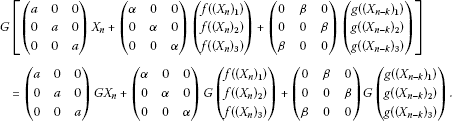Hence, the system (2.1) is ${Z}_{3}$ equivariant. Let $T=\frac{2\pi }{{\omega }_{1}}$ or $T=\frac{2\pi }{{\omega }_{2}}$. Consider the subgroup of ${Z}_{3}×{S}^{1}$: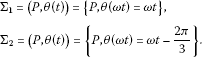It is clear that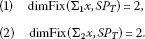The system (2.1) is equivariant with respect to the ${Z}_{3}$-action where the subgroups ${\mathrm{\Sigma }}_{1}$ and ${\mathrm{\Sigma }}_{2}$ of ${Z}_{3}$ act by synchronization and by permutation.

Theorem 3.1 Assume that (H1)-(H3) hold.

1. (1)

${\beta }_{1j}$ is defined as in (2.4). When near $\beta ={\beta }_{1j}$, there exist synchronous periodic solutions of a period ${P}_{T}$ near $\frac{2\pi }{{\omega }_{1}}$, bifurcated simultaneously from the zero solution of the system (2.1).

2. (2)

${\beta }_{2j}$ is defined as in (2.6). When near $\beta ={\beta }_{2j}$, there exist phase-locked periodic solutions of a period ${P}_{T}$ near $\frac{2\pi }{{\omega }_{2}}$, bifurcated simultaneously from the zero solution of the system (2.1).

4 Direction and stability of the Hopf bifurcation in a discrete neural network model

In this section, we give some results of direction and stability of the Hopf bifurcation in a discrete neural network model. The method we used is based on the theories of a discrete system by Kuznetsov . Throughout this section, we always assume that the system (2.1) undergoes the Hopf bifurcation at the origin $\left(0,0,0\right)$ for $\beta ={\beta }_{lj}$, $l=1,2$ and then ${e}^{±i{\omega }_{1,2}}$ are corresponding roots with modulus one of the characteristic equation at the origin.

Without loss of generality, denote any one of these the critical values ${\beta }_{lj}$ ($l=1,2$, $j=0,1,2,\dots ,k-1$) by ${\beta }_{\ast }$ at which the system (2.1) undergoes the Hopf bifurcation from $\left(0,0,0\right)$ and ${\omega }_{1,2}$ by ${\omega }_{\ast }$. Rewritting $\beta ={\beta }_{\ast }+\mu$, then $\mu =0$ is Hopf bifurcation value of Eq. (2.1).

For the map (2.1), we have

${Y}_{n+1}=A{Y}_{n}+\frac{1}{2}B\left({Y}_{n},{M}_{n}\right)+\frac{1}{6}C\left({Y}_{n},{Y}_{n},{Y}_{n}\right)+O\left({\parallel {Y}_{n}\parallel }^{4}\right),$

where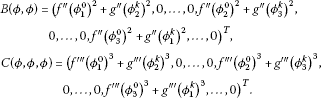Let $q\in {C}^{3\left(k+1\right)}$ be a complex eigenvector corresponding to ${e}^{i{\omega }_{\ast }}$ satisfying

$Aq={e}^{i{\omega }_{\ast }}q,\phantom{\rule{2em}{0ex}}A\overline{q}={e}^{-i{\omega }_{\ast }}\overline{q}.$

We also introduce an adjoint eigenvector ${q}^{\ast }\in {C}^{3\left(k+1\right)}$ having the properties

${A}^{T}q\ast ={e}^{-i{\omega }_{\ast }}{q}^{\ast },\phantom{\rule{2em}{0ex}}A\overline{q}\ast ={e}^{i{\omega }_{\ast }}{\overline{q}}^{\ast },$

and satisfying the normalization

$〈{q}^{\ast },q〉=1.$

Using Theorem 2.1 of Ref. , we obtain

$q={\left({q}_{0}^{T},{e}^{\frac{i2\pi }{3}}{q}_{0}^{T},{e}^{\frac{i4\pi }{3}}{q}_{0}^{T}\right)}^{T}$

and

${q}^{\ast }={\left({q}_{0}^{\ast T},{e}^{\frac{i2\pi }{3}}{q}_{0}^{\ast T},{e}^{\frac{i4\pi }{3}}{q}_{0}^{\ast T}\right)}^{T},$

where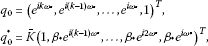and

$K={\left[{\overline{q}}_{0}^{\ast T}\cdot {q}_{0}+{\overline{q}}_{0}^{\ast T}B{e}^{-\frac{\left(k+1\right)i{\omega }^{\ast }}{k}}{q}_{0}\right]}^{-1}.$

Now, we begin to analyze Eq. (2.1). Let ${T}^{c}$ denote a real eigenspace corresponding to ${e}^{±i{\omega }^{\ast }}$, which is two-dimensional and is spanned by $\left\{Re\left(q\right),Im\left(q\right)\right\}$, and ${T}^{s}$ be a real eigenspace corresponding to all eigenvalues of ${A}^{T}$ other than ${e}^{±i{\omega }^{\ast }}$ which is $3\left(k+1\right)$-dimensional.

For any $x\in {R}^{3\left(k+1\right)}$, we have its decomposition

$x=zq+\overline{z}\overline{q}+y,$

where $z\in C$, $zq+\overline{z}\overline{q}\in {T}^{c}$, $y\in {T}^{s}$. The complex variable z can be viewed as a new coordinate on ${T}^{c}$, and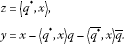In the coordinate, the map at $\mu =0$ has the form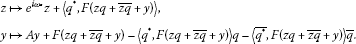Using the Taylor expansions,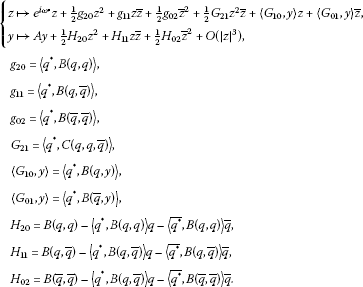(4.1)

Now, we seek the center manifold which has the representation

$y=V\left(z,\overline{z}\right)=\frac{1}{2}{w}_{20}{z}^{2}+{w}_{11}z\overline{z}+\frac{1}{2}{w}_{02}{\overline{z}}^{2}+O\left({|z|}^{3}\right),$
(4.2)

where $〈{q}^{\ast },{w}_{ij}〉=0$. Substituting (4.2) into (4.1), we have

$\left\{\begin{array}{c}{w}_{20}={\left({e}^{i2{\omega }_{\ast }}I-A\right)}^{-1}{H}_{20},\hfill \\ {w}_{11}={\left(I-A\right)}^{-1}{H}_{11}=0,\hfill \\ {w}_{02}={\left({e}^{-i2{\omega }_{\ast }}-A\right)}^{-1}{H}_{02}=0.\hfill \end{array}$

We restrict (4.1) to the center manifold, up to a cubic term, then we have

$\begin{array}{rcl}{g}_{21}& =& 〈{q}^{\ast },C\left(q,q,\overline{q}\right)〉-2〈{q}^{\ast },B\left(q,{\left(I-A\right)}^{-1}\right)B\left(q,\overline{q}\right)〉\\ +〈{q}^{\ast },B{\left(\overline{q},\left({z}^{2}I-A\right)\right)}^{-1}B\left(q,q\right)〉-\frac{1-2z}{{z}^{2}-z}〈{q}^{\ast },B\left(q,q\right)〉〈{q}^{\ast },B\left(q,\overline{q}\right)〉\\ -2{\left(1-\overline{z}\right)}^{-1}{|〈{q}^{\ast },B\left(q,\overline{q}\right)〉|}^{2}-{\left({z}^{2}-\overline{z}\right)}^{-1}{|〈{q}^{\ast },B\left(\overline{q},\overline{q}\right)〉|}^{2}.\end{array}$

Define

${c}_{1}\left(\beta \right)=\frac{{g}_{20}{g}_{11}\left(2z+\overline{z}-3\right)}{2\left(\overline{z}-1\right)\left({z}^{2}-z\right)}+\frac{{|{g}_{11}|}^{2}}{\left(1-\overline{z}\right)}+\frac{{|{g}_{02}|}^{2}}{2\left({z}^{2}-\overline{z}\right)}+\frac{{g}_{21}}{2}.$

We have Theorem 4.1.

Theorem 4.1 The direction and stability of the Hopf bifurcation in the map (2.1) is determined by the sign of $v=-\frac{Re\left[{e}^{-i{\omega }_{\ast }}{c}_{1}\left({\beta }_{\ast }\right)\right]}{\frac{d{|\lambda |}^{2}}{d\beta }{|}_{\beta ={\beta }_{\ast }}}$: if $v>0$ (<0), then the Hopf bifurcation is supercritical (subcritical).

5 Computer simulation

To illustrate analytical results, let us consider the following example:

$\left\{\begin{array}{c}{x}_{n+1}=a{x}_{n}+\alpha tanh\left({x}_{n}\right)+\beta tanh\left({y}_{n-2}\right),\hfill \\ {y}_{n+1}=a{y}_{n}+\alpha tanh\left({y}_{n}\right)+\beta tanh\left({z}_{n-2}\right),\hfill \\ {z}_{n+1}=a{z}_{n}+\alpha tanh\left({z}_{n}\right)+\beta tanh\left({x}_{n-2}\right).\hfill \end{array}$
(5.1)

Firstly, we choose parameters such that Eq. (2.5) is met. When $a=0.3$, $\alpha =-1$, $\beta =-0.3$, we have the result that a period 2 solution appears. See Figure 1.

Next, one possible choice of the parameters is $a=0.8$, $\alpha =-0.3$, $\beta =-0.5$. In this case, the zero solution is asymptotically stable. See Figure 2.

Finally, the parameters are $a=0.8$, $\alpha =-0.3$, $\beta =-0.535$. In this case, multiple branches of periodic solutions appear. These solutions are shown in Figure 3.

It is shown that in Figures 1, 2 and 3, for different values of parameters, the system (5.1) exhibits its different dynamics. At first, the trivial solution is stable, then it loses its stability, and several different periodic patterns by a discrete Hopf bifurcation can be observed, which depend on different values of parameters.

References

1. 1.

Golubitsky M, Stewart IN, Schaeffer DG Appl. Math. Sci. 69. In Singularities and Groups in Bifurcation Theory: Vol. 2. Springer, New York; 1988.

2. 2.

Hopfield J: Neurons with graded response have collective computational properties like two-state neurons. Proc. Natl. Acad. Sci. USA 1984, 81: 3088–3092. 10.1073/pnas.81.10.3088

3. 3.

Campbell SA, Ncube I, Wu J: Multistability and stable asynchronous periodic oscillations in a multiple-delayed neural system. Physica D 2006, 214: 101–119. 10.1016/j.physd.2005.12.008

4. 4.

Wu J: Symmetric functional differential equations and neural networks with memory. Trans. Am. Math. Soc. 1998, 350: 4799–4838. 10.1090/S0002-9947-98-02083-2

5. 5.

Li L, Yuan Y: Dynamics in three cells with multiple time delays. Nonlinear Anal., Real World Appl. 2008, 9: 725–746. 10.1016/j.nonrwa.2006.12.015

6. 6.

Lin J, Cheng S: Doubly periodic traveling waves in a cellular neural network with linear reaction. Adv. Differ. Equ. 2009., 2009: Article ID 243245

7. 7.

Zhang C, Zheng B: Stability and bifurcation of a two-dimension discrete neural network model with multi-delays. Chaos Solitons Fractals 2007, 31: 1232–1242. 10.1016/j.chaos.2005.10.074

8. 8.

Guo S, Huang L, Wang L: Exponential stability of discrete-time Hopfield neural networks. Comput. Math. Appl. 2004, 47: 1249–1256. 10.1016/S0898-1221(04)90119-8

9. 9.

Chossat P, Golubitsky M: Iterates of maps with symmetry. SIAM J. Math. Anal. 1998, 19: 1259–1270.

10. 10.

Zhang C, Zheng B, Zhang Y: A model in a coupled system of simple neural oscillators with delays. J. Comput. Appl. Math. 2009, 229: 616–622.

11. 11.

Kuznetsov YA: Elements of Applied Bifurcation Theory. 3rd edition. Springer, New York; 2004.

Acknowledgements

The authors are very grateful to the referees for their valuable suggestions. This work was supported by the National Natural Science Foundation of China (No. 10871056) and the Heilongjiang Province Science Foundation for Youths (No. QC2009C100).

Author information

Authors

Corresponding author

Correspondence to Chunrui Zhang.

Competing interests

The authors declare that they have no competing interests.

Authors’ contributions

The authors have achieved equal contributions to each part of this paper. All the authors read and approved the final manuscript.

Authors’ original submitted files for images

Below are the links to the authors’ original submitted files for images.

Rights and permissions

Reprints and Permissions

Wang, X., Zhang, C. Symmetry discrete-time delayed neural network. Adv Differ Equ 2012, 207 (2012). https://doi.org/10.1186/1687-1847-2012-207

• Accepted:

• Published:

• DOI: https://doi.org/10.1186/1687-1847-2012-207

Keywords

• discrete neural network
• stability
• Hopf bifurcation
• multiple periodic solutions